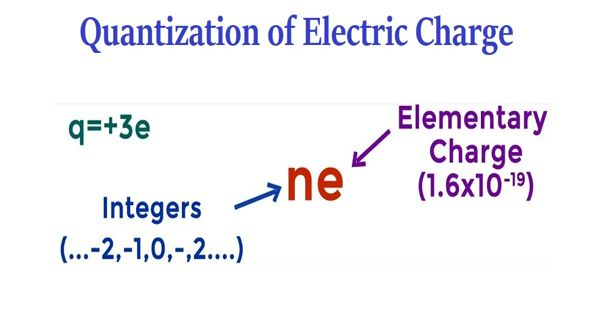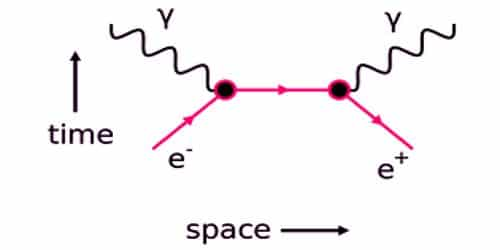# Quantization of Electric Charge

Quantization of Electric Charge

Electric charge is the transfer of electrons from one material to another. Experimentally it has been known for a long time that the electric charges of the observed particles appear to be quantized. The fundamental unit of electric charge (e) is the charge carried by the electron and its unit is the coulomb. ‘e’ has the magnitude 1.6 × 10-19 C. That is to say, the observed value of electric charge (q) of a particle will be integral multiples of (e) 1.6×10-19 coulombs. e = 1.6 × 10-19 C is the magnitude of the lowest possible charge which is carried by an electron and proton. The cause of the quantization of electric charge is due to the fact that when one body is rubbed with the other, an integral number of electrons are transferred. The basic cause of quantization of electric charge is that during rubbing only an integral number of electrons can be transferred from one body to another. Electric charge is a fundamental property of a particle just like its mass and length. It also signifies the force of attraction between two electrons.

Quantization of charge means that when we say something has a given charge, we mean that that is how many times the charge of a single electron it has. It implies that charge can assume only certain discrete values. There is no scientific explanation for the quantization of electric charge in electrodynamics theory and modern physics but it can be verified experimentally. This approach uses the allowed invariances of the Lagrangian and their associated anomaly cancellation equations.Fig:  Quantization of electric charge and the principle of conservation

Principle of Conservation of Charges: “Electric charge can neither be created nor be destroyed but it is transferred from one part of a system to another part of the system so that the total charge of an isolated system remains constant.” The total charge in an isolated system is always conserved. When there are many bodies in an isolated system, the charges get transferred from one body to another but the net charge of the system remains the same.

In nature, the electric charge of any system is always an integral multiple of the least amount of charge. Because all charges are associated with a whole electron, this is possible. During rubbing or natural forces, no new charge is created. The charges are either redistributed or a neutron breaks up into proton and electron of equal and opposite charge. It means that the quantity can take only one of the discrete set of values. The charge is denoted by the symbol ‘q’ and its standard unit is Coulomb. Mathematically charge is the number of electrons multiplied by the charge on 1 electron. That is: q = ne. The charge, q = ne where n is an integer. i.e.,

q = ne where n = 0, 1, 2,… (Both positive and negative integers)

The charge cannot assume any value between the integers. Charges have no direction but can be positive or negative.